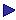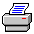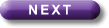Tutorial Contents Tutorial Eight: Relations- Reflexivity - Symmetry -Transitivity - Connectedness - Example of a tableau proof - Eqivalent Relations More Tutorials OneTwoThreeFourFiveSixEquivalence relations

An equivalence relation is a relation which is reflexive, symmetric and transitive.

If represented graphically, its domain divides into different parts such that:

1. there is no arrow from a dot in one part to a dot in another, and

2. every dot is connected to every dot (including itself) in the same part.

The parts of the domain (properly speaking, the sub-sets of the domain) are equivalence classes.

An example of an equivalence relation is "x is a member of the same college as y" in the domain of Oxford undergraduates. The corresponding equivalence classes will be the set of the members of Brasenose, the set of the members of Christ Church, and so on.

Equivalence relations have a number of uses; in particular it is possible to define certain abstract entities in terms of them.

Consider, for example, the relation expressed by "x is neither older nor younger than y": let us abbreviate it as "Nxy", and call the relation expressed by it N.

Now N is an equivalence relation in the domain of living things. So "Nxy" is equivalent to "x belongs to (has) the same equivalence class of N as y". But also, of course "Nxy" is equivalent to "x is (has) the same age as y".

So, to have a certain age is just to belong to a certain equivalence class of N.

So, one could think of "age" as defined in terms of N. In that case age is an abstraction of N.

This is a trivial example; and no one would think of defining age in this way  not least because one must first discover two people's ages before one can say whether "Nxy" is true of them. However it would be more worthwhile to try to define "liquid capacity" in terms of  "x holds the same as y", since one can test for this by pouring liquid from one container into another. (If you prefer, one could try to define "liquid capacity" in terms of "it is possible to fill x and to pour its contents into y without spilling and it is possible to fill y and to pour its contents into x without spilling".)

Or one might try to define hardness in minerals in terms of "x and y will not scratch each other". But of course, for either of these definitions to work the relations which are chosen must actually be equivalence relations in the appropriate domains. And I believe that the relation expressed by "x and y will not scratch each other" is not an equivalence relation in the domain of minerals. (See Hodges, Section 33 for more discussion on this topic.)Print this page